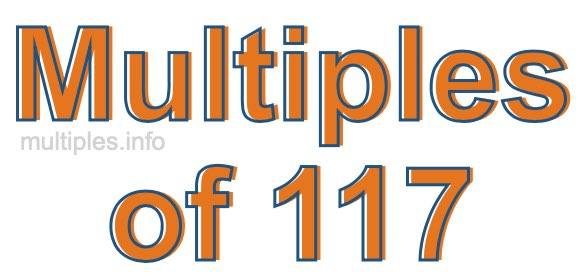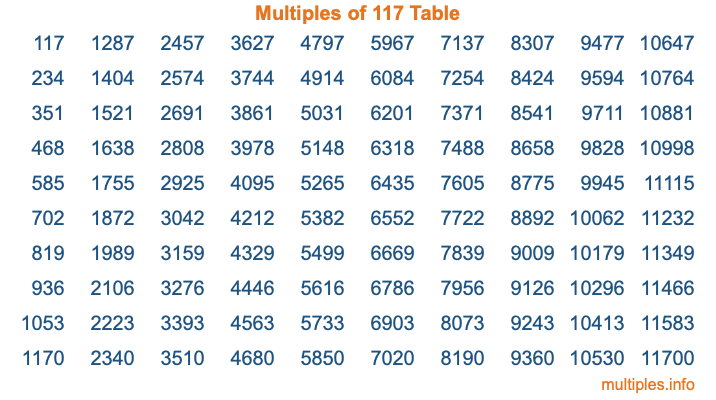Multiples of 117Welcome to the Multiples of 117 page. Here we will first teach you everything you will ever need to know about the multiples of 117, and then give you a study guide summary of everything we taught you to make sure you remember it all. Use this page to look up facts and learn information about the multiples of 117. This page will make you a multiples of one hundred seventeen expert!

Definition of Multiples of 117
Multiples of 117 are all the numbers that when divided by 117 equal an integer. Each of the multiples of 117 are called a multiple. A multiple of 117 is created by multiplying 117 by an integer.

Therefore, to create a list of multiples of 117, you start with 1 multiplied by 117, then 2 multiplied by 117, then 3 multiplied by 117, and so on for as long as you want. Thus, the list of the first five multiples of 117 is 117, 234, 351, 468, and 585. To see a larger list of multiples of 117, see the printable image of Multiples of 117 further down on this page. We also have a category where you can choose any nth multiple of 117.

Multiples of 117 Checker
The Multiples of 117 Checker below checks to see if any number of your choice is a multiple of 117. In other words, it checks to see if there is any number (integer) that when multiplied by 117 will equal your number. To do that, we divide your number by 117. If the the quotient is an integer, then your number is a multiple of 117.

Is  a multiple of 117?

Least Common Multiple of 117 and ...
A Least Common Multiple (LCM) is the lowest multiple that two or more numbers have in common. This is also called the smallest common multiple or lowest common multiple and is useful to know when you are adding our subtracting fractions. Enter one or more numbers below (117 is already entered) to find the LCM.

Check out our LCM Calculator if you need more details about the Least Common Multiple or if you need the LCM for different numbers for adding and subtraction fractions.

nth Multiple of 117
As we stated above, 117 is the first multiple of 117, 234 is the second multiple of 117, 351 is the third multiple of 117, and so on. Enter a number below to find the nth multiple of 117.

th multiple of 117

Multiples of 117 vs Factors of 117
117 is a multiple of 117 and a factor of 117, but that is where the similarities end. All postive multiples of 117 are 117 or greater than 117. All positive factors of 117 are 117 or less than 117.

Below is the beginning list of multiples of 117 and the factors of 117 so you can compare:

Multiples of 117: 117, 234, 351, 468, 585, etc.

Factors of 117: 1, 3, 9, 13, 39, 117

As you can see, the multiples of 117 are all the numbers that you can divide by 117 to get a whole number. The factors of 117, on the other hand, are all the whole numbers that you can multiply by another whole number to get 117.

It's also interesting to note that if a number (x) is a factor of 117, then 117 will also be a multiple of that number (x).

Multiples of 117 vs Divisors of 117
The divisors of 117 are all the integers that 117 can be divided by evenly. Below is a list of the divisors of 117.

Divisors of 117: 1, 3, 9, 13, 39, 117

The interesting thing to note here is that if you take any multiple of 117 and divide it by a divisor of 117, you will see that the quotient is an integer.

Multiples of 117 Table
Below is an image of the first 100 multiples of 117 in a table. The table is in chronological order, column by column. The first column has the first ten multiples of 117, the second column has the next ten multiples of 117, and so on.The Multiples of 117 Table is also referred to as the 117 Times Table or Times Table of 117. You are welcome to print out our table for your studies.

Negative Multiples of 117
Although not often discussed or needed in math, it is worth mentioning that you can make a list of negative multiples of 117 by multiplying 117 by -1, then by -2, then by -3, and so on, to get the following list of negative multiples of 117:

-117, -234, -351, -468, -585, etc.

Multiples of 117 Summary
Below is a summary of important Multiples of 117 facts that we have discussed on this page. To retain the knowledge on this page, we recommend that you read through the summary and explain to yourself or a study partner why they hold true.

There are an infinite number of multiples of 117.

A multiple of 117 divided by 117 will equal a whole number.

117 divided by a factor of 117 equals a divisor of 117.

The nth multiple of 117 is n times 117.

The largest factor of 117 is equal to the first positive multiple of 117.

117 is a multiple of every factor of 117.

117 is a multiple of 117.

A multiple of 117 divided by a divisor of 117 equals an integer.

117 divided by a divisor of 117 equals a factor of 117.

Any integer times 117 will equal a multiple of 117.

Multiples of a Number
Here you can get the multiples of another number, all with the same attention to detail as we did for multiples of 117 on this page.

Multiples of
Multiples of 118
Did you find our page about multiples of one hundred seventeen educational? Do you want more knowledge? Check out the multiples of the next number on our list!